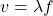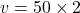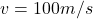## PLEASE HELP ME!!!! I’LL MARK YOU BRAINLIEST IF YOU ANSWER THIS CORRECTLY!!!!! I NEED THESE DONE TODAY!!!! The speed of sound in

Question

PLEASE HELP ME!!!! I’LL MARK YOU BRAINLIEST IF YOU ANSWER THIS CORRECTLY!!!!! I NEED THESE DONE TODAY!!!!

The speed of sound in air at 20 deg C. is approximately 340 m/s

True
False
Wave speed = wave frequency x wave length

True
False

Because f=1/period, wave speed = wavelength/period

True
False

What is the wavelength of a sound wave that has a speed of 340m/s and a frequency of 440 hz

0.773 m

149,600 m

1.29 m

What is the wave speed of a wave that has a frequency of 50 Hz and a wavelength of 2.0 m?

25 m/s

100 m/s

0.04 m/s

in progress 0
5 months 2021-08-24T13:03:39+00:00 1 Answers 3 views 0

## v = 100 m/s

Explanation:

1)

As we know by the formula of speed of sound we have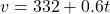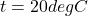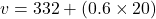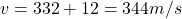So approx speed of the sound is nearly 340 m/s

so its TRUE

2)

Wave speed is given as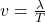so it is given as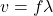TRUE

3)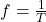so from above equation we have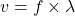4)

Wavelength is given as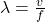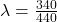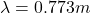5)

As we know that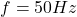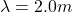so we will have